JEE  >  Test: Distribution Of Random Variables

# Test: Distribution Of Random Variables

Test Description

## 10 Questions MCQ Test Mathematics (Maths) Class 12 | Test: Distribution Of Random Variables

Test: Distribution Of Random Variables for JEE 2023 is part of Mathematics (Maths) Class 12 preparation. The Test: Distribution Of Random Variables questions and answers have been prepared according to the JEE exam syllabus.The Test: Distribution Of Random Variables MCQs are made for JEE 2023 Exam. Find important definitions, questions, notes, meanings, examples, exercises, MCQs and online tests for Test: Distribution Of Random Variables below.
Solutions of Test: Distribution Of Random Variables questions in English are available as part of our Mathematics (Maths) Class 12 for JEE & Test: Distribution Of Random Variables solutions in Hindi for Mathematics (Maths) Class 12 course. Download more important topics, notes, lectures and mock test series for JEE Exam by signing up for free. Attempt Test: Distribution Of Random Variables | 10 questions in 10 minutes | Mock test for JEE preparation | Free important questions MCQ to study Mathematics (Maths) Class 12 for JEE Exam | Download free PDF with solutions
 1 Crore+ students have signed up on EduRev. Have you?
Test: Distribution Of Random Variables - Question 1

### If P (A) = 0.8, P (B) = 0.5 and P(B|A) = 0.4, find P(A ∩ B)

Detailed Solution for Test: Distribution Of Random Variables - Question 1

We have ,
P (A) = 0.8, P (B) = 0.5 and P(B|A) = 0.4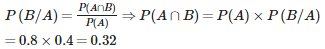Test: Distribution Of Random Variables - Question 2

### If S is the sample space associated with a random experiment. Then a real valued function which assign to each element of S, a unique real number is called a ……​

Test: Distribution Of Random Variables - Question 3

### Random variable is a real valued function whose domain is the sample space of a ……… and range is the set of ………​

Detailed Solution for Test: Distribution Of Random Variables - Question 3

A random variable is a numerical description of the outcome of a statistical experiment. It can be any outcome from some chance process, like how many heads will occur in a series of 20 flips etc. So as it is related to the chance of an event. Therefore its domain is the sample space of random experiment and as chance of occuring can never be a fraction or a negative number. So range is the set of real numbers.

Test: Distribution Of Random Variables - Question 4

The probability distribution of the number of heads in three tosses of a coin is:

Test: Distribution Of Random Variables - Question 5

A random variable X has the following probability distribution: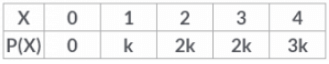Then k =?​

Detailed Solution for Test: Distribution Of Random Variables - Question 5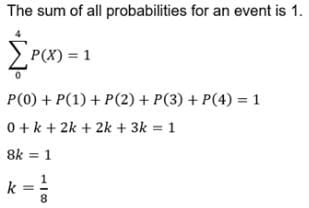Test: Distribution Of Random Variables - Question 6

An urn contains 3 red and 2 black balls. Two balls are randomly drawn. Let X represents the number of black balls. The possible values of X are:​

Detailed Solution for Test: Distribution Of Random Variables - Question 6

The number of ways in which two balls can be represented are {(RR), (RB), (BR), (BB)}
Let X represent the number of black balls. Possible values of X are:
X (RR) = 0, X (RB) = 1,
X(BR) 1 and X (BB) = 2.
Therefore the possible values of X are 0, 1 and 2.

Test: Distribution Of Random Variables - Question 7

A random variable X has the probability distribution: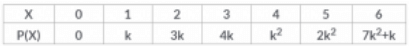Then the value of k is:​

Detailed Solution for Test: Distribution Of Random Variables - Question 7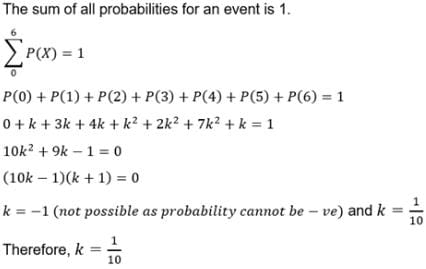Test: Distribution Of Random Variables - Question 8

If a random variable X assumes all possible values in a given interval, then it is called ………​

Detailed Solution for Test: Distribution Of Random Variables - Question 8

As we known that a random variable which can assume all possible values within a given interval is called a continuous random variable.

Test: Distribution Of Random Variables - Question 9

A random variable X has the probability distribution: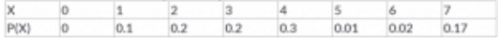Then the value P(X ≥ 5) is:​

Detailed Solution for Test: Distribution Of Random Variables - Question 9

P(5) = 0.01, P(6) = 0.02, P(7) = 0.17
P(X ≥ 5) = P(5) + P(6) + P(7)
= 0.01 + 0.02 + 0.17
= 0.20

Test: Distribution Of Random Variables - Question 10

If a random variable X assume values x1, x2, x3, …, xn with the corresponding probabilities p1, p2, p3, …, pn. Then

## Mathematics (Maths) Class 12

209 videos|218 docs|139 tests
Information about Test: Distribution Of Random Variables Page
In this test you can find the Exam questions for Test: Distribution Of Random Variables solved & explained in the simplest way possible. Besides giving Questions and answers for Test: Distribution Of Random Variables, EduRev gives you an ample number of Online tests for practice

## Mathematics (Maths) Class 12

209 videos|218 docs|139 tests(Scan QR code)